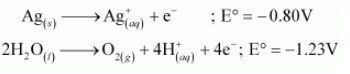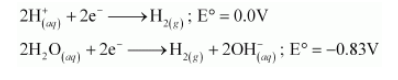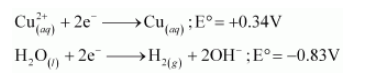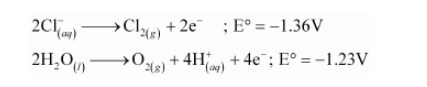# Predict the products of electrolysis in each of the following:

Question:

Predict the products of electrolysis in each of the following:

(i) An aqueous solution of $\mathrm{AgNO}_{3}$ with silver electrodes

(ii) An aqueous solution $\mathrm{AgNO}_{3}$ with platinum electrodes

(iii) A dilute solution of $\mathrm{H}_{2} \mathrm{SO}_{4}$ with platinum electrodes

(iv) An aqueous solution of $\mathrm{CuCl}_{2}$ with platinum electrodes.

Solution:

(i) $\mathrm{AgNO}_{3}$ ionizes in aqueous solutions to form $\mathrm{Ag}^{+}$and $\mathrm{NO}_{3}^{-}$ions.

On electrolysis, either $\mathrm{Ag}^{+}$ions or $\mathrm{H}_{2} \mathrm{O}$ molecules can be reduced at the cathode. But the reduction potential of $\mathrm{Ag}^{+}$ions is higher than that of $\mathrm{H}_{2} \mathrm{O}$.Hence, $\mathrm{Ag}^{+}$ions are reduced at the cathode. Similarly, $\mathrm{Ag}$ metal or $\mathrm{H}_{2} \mathrm{O}$ molecules can be oxidized at the anode. But the oxidation potential of $\mathrm{Ag}$ is higher than that of $\mathrm{H}_{2} \mathrm{O}$ molecules.Therefore, Ag metal gets oxidized at the anode.

(ii) Pt cannot be oxidized easily. Hence, at the anode, oxidation of water occurs to liberate O2. At the cathode, Ag+ ions are reduced and get deposited.

(iii) $\mathrm{H}_{2} \mathrm{SO}_{4}$ ionizes in aqueous solutions to give $\mathrm{H}^{+}$and $\mathrm{SO}_{4}^{2-}$ ions.

$\mathrm{H}_{2} \mathrm{SO}_{4(a q)} \longrightarrow 2 \mathrm{H}_{(a q)}^{+}+\mathrm{SO}_{4}^{2-}(a q)$

On electrolysis, either of $\mathrm{H}^{+}$ions or $\mathrm{H}_{2} \mathrm{O}$ molecules can get reduced at the cathode. But the reduction potential of $\mathrm{H}^{+}$ions is higher than that of $\mathrm{H}_{2} \mathrm{O}$ molecules.Hence, at the cathode, H+ ions are reduced to liberate H2 gas.

On the other hand, at the anode, either of $\mathrm{SO}_{4}^{2-}$ ions or $\mathrm{H}_{2} \mathrm{O}$ molecules can get oxidized. But the oxidation of $\mathrm{SO}_{4}^{2-}$ involves breaking of more bonds than that

of $\mathrm{H}_{2} \mathrm{O}$ molecules. Hence, $\mathrm{SO}_{4}^{2-}$ ions have a lower oxidation potential than $\mathrm{H}_{2} \mathrm{O} .$ Thus, $\mathrm{H}_{2} \mathrm{O}$ is oxidized at the anode to liberate $\mathrm{O}_{2}$ molecules.

(iv) In aqueous solutions, $\mathrm{CuCl}_{2}$ ionizes to give $\mathrm{Cu}^{2+}$ and $\mathrm{Cl}^{-}$ions as:

$\mathrm{CuCl}_{2(\text { aq })} \longrightarrow \mathrm{Cu}_{(\text {(aq) })}^{2+}+2 \mathrm{Cl}_{(\text {aq })}^{-}$

On electrolysis, either of $\mathrm{Cu}^{2+}$ ions or $\mathrm{H}_{2} \mathrm{O}$ molecules can get reduced at the cathode. But the reduction potential of $\mathrm{Cu}^{2+}$ is more than that of $\mathrm{H}_{2} \mathrm{O}$ molecules.Hence, $\mathrm{Cu}^{2+}$ ions are reduced at the cathode and get deposited

Similarly, at the anode, either of $\mathrm{Cl}^{-}$or $\mathrm{H}_{2} \mathrm{O}$ is oxidized. The oxidation potential of $\mathrm{H}_{2} \mathrm{O}$ is higher than that of $\mathrm{Cl}^{-}$.But oxidation of $\mathrm{H}_{2} \mathrm{O}$ molecules occurs at a lower electrode potential than that of $\mathrm{Cl}^{-}$ions because of over-voltage (extra voltage required to liberate gas). As a result, $\mathrm{Cl}^{-}$ions are oxidized at the anode to liberate $\mathrm{Cl}_{2}$ gas.